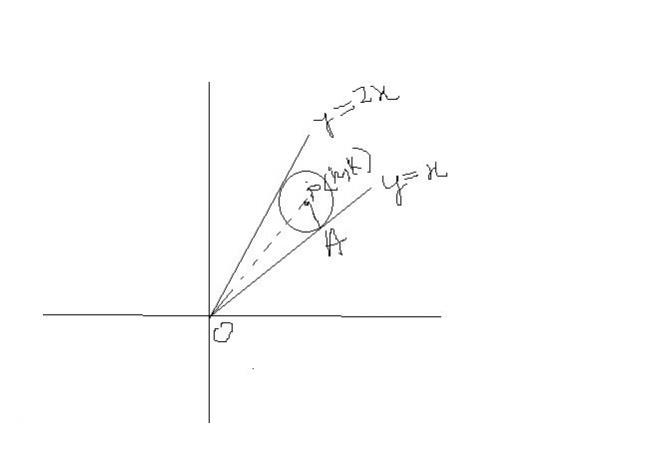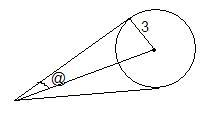# Find the length of OA

Let 2x2+y2-3xy=0 be the equation of a pair of tangents from origin O to a circle of radius 3 with center in the first quadrant. If A is one of the points of contact, Find the length OA

•rkrish ·

Ans : 3(âˆš10 + 3)

•skygirl ·

k the esiest one :Pnow |k-2h| =3âˆš5 , |k-h|=3âˆš2

solving this we get P.

then OA2=OP2-PA2

•Optimus Prime ·

2x2+y2-3xy=0
i.e (y-x)(y-2x)=0
lines are y=x and y=2x

let C(a,b) be the center of the circle whose tangents are two lines y=x and y=2x

clearly b>a and b<2a

radius=3=length of perpendicular from C on the two tangents

=b-a/√2 = 2a-b/√5

from above, a=3(√5+√2) and b = 3(√5+2√2)

=(a2+b2-9
=9(7+2√10)+9(5+8+4√10)-9
=171+54√10=90+81+2√90x81=(√90+√81)2
therefroe OA=9+√90=9+3√10

•rkrish ·Angle b/w tngnts = 2@

tan 2@ = 2âˆš9/4 - 2 /(3) = 1/3
So, tan @ = âˆš10 - 3

Also tan @ = 3/oa
So,oa = 3/(âˆš10 - 3) = 3(âˆš10 + 3)

•eureka123 ·

wow.....three different methods..........

•shanu kumar ·

let C(a,b) be the center of the circle whose tangents are two lines y=x and y=2xclearly b>a and b<2a

Plz explain this

•